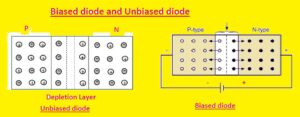Hello, readers welcome to the new post. In this post, we will discuss Forward biased p-n junction diode. The diode is an electronic device that is used in electronic projects for the conversion of ac to dc the process of ac to dc conversion is called rectification and the circuit used is the rectifier. A diode is two terminal devices anode and a cathode, the positive terminal is called the anode and the negative is called the cathode.

The process through which power is given to the diode is called biasing. That has two types forward biased and reversed-biased. In this post, we will discuss the forward biasing of the PN Junction diode. So let’s get started.

### What is Forward Bias?

• During the forward biasing state of the diode P side or positive terminal of the diode is attached to the positive pin of the battery and the negative terminal or cathode of the diode is connected to the negative side of the battery.
• The voltage given is reversed to the potential barrier junction. In a result potential barrier and junction area reduces and the majority of carriers passing through the junction of the diode
• Through the quantity of voltage needed is less than the total reduction of the barrier. So the width of the depletion layer is reduced.
•  The number of electrons and holes combined after crossing the junction. Every hole in the P region combines with an electron which is in the N region.
• As a result, covalent bonds destroy, and electrons in larger quantities are produced from the breakage of covalent bonds and goest to the anode.

### Forward biased p-n junction diode

• Forward biased is type of diode biasing in which curent flows when the power source is connected to the diode. During forward biased diode P pin od diode is attached to the positive terminal of the battery and n region to the negative terminal
• If there is no voltage source connected to the diode no curent flows through it. If the voltage given increases to 0.1 volts then the depletion region of the diode decreases. As a result, a small curent flows through the PN Junction diode.
• As it is very less curent that is considered as negligible
• If the voltage given on the PN junction diode is increased more then further electrons and holes are generated in the diode. That causes in decrement of the depletion region
• With an increment of electrons and holes, the current increases according to the voltage applied.### What is Electron Current

• Let’s suppose that we provide 0.7 volts at the input to the diode of silicon material and 0.3 for the germanium diode then on the junction diode has current flow.
• In this state negative point of the battery generates larger free electrons to the N region and moves to P region combining to holes.
• So larger electrons are created at the negative terminal and holes combining this election at the negative point of the battery
• Due to movement of electrons generated to the negative end produces the electrical field. The field direction is reversed to the direction of the positive field existing at deletion that has positive ions.
• As there is a large number of electrons at the N region they have the force of repulsion from their neighboring electrons and move to the high to low concentration side or P region.  Some electrons merge to holes before crossing the depletion region  in this way electrons disappeared

• The ion that get the electrons become a neutral atom. So depletion region at N region close to the PN junction decreases.
• The remaining electrons cross the depletion region and go to P region. Free electrons that cross depletion regions have larger holes in P regions and move to the positive terminal of the source.
• So free election that crosses the depletion region causes the current flow from one point  to another of diode that current called electron current### What is Hole Current

• The positive terminal of the battery provides larger holes at the P region and get electrons from the N region of the diode. In simple words, holes staring from the positive terminal and electrons end at the positive terminal by combining with holes
• The holes that start from a positive point generate a larger positive electric field at the P region. That field has a direction opposite to the negative field of negative ions at the depletion region
• Larger holes at the P region have repulsion forces at each other and move to low concentrations of holes or the N region that has electrons.
• So they cross the depletion region and some during this crossing combine with negative ions depletion region and merge with them and get disappeared and create a neutral atom
• So negative in P region reduce and completely diminished
• The other holes cross the depletion region and attract the negative terminal of the battery and these holes cause the curent flow in the diode called hole current.

That is all about the Forward biased p-n junction diode all details and circuits have been explained if you have any questions ask here.